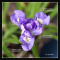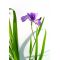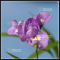##### The Japanese Bonsai specialist
Direct order Contact Help / Services Newsletter# Iris gracilipes purple dwarf variety

Iris gracilipesref. : 65058,40

This item is temporarily unavailableNotify availability

###### Description

Rare miniature iris gracilipes, vivacious plant, rhizomes. Sold with a plastic pot.

The foliage is similar to that of the common iris and at the beginning of summer a flower stem appears, blooming into small purple flowers. An ideal kusamono pot plant or it can be planted in a flowerbed.

Type: Herbaceous perennial

Family: Iridaceae

Flower Colour: Blue

Flowering Season: Summer

Size: Leaves 90 / 100 mm long and 3 to 5 mm wide.

Origin: Asia

Preferred Conditions: Moist, well drained soil

Preferred Aspect: Full sun

#iris 4.4 #gracilipes 4 #kusamono 3.8 #purple 3.6 #small 3.5 #preferred 2.9 #variety 2.7 #summer 2.6 #plants 2.6 #flower 2.6

Formule
(( ROUND((CHAR_LENGTH(b.article_nom)-CHAR_LENGTH(REPLACE(b.article_nom, 'iris', '')))/LENGTH('iris')) + ROUND((CHAR_LENGTH(b.article_description)-CHAR_LENGTH(REPLACE(b.article_description, 'iris', '')))/LENGTH('iris')) ) * 4.4) + (( ROUND((CHAR_LENGTH(b.article_nom)-CHAR_LENGTH(REPLACE(b.article_nom, 'gracilipes', '')))/LENGTH('gracilipes')) + ROUND((CHAR_LENGTH(b.article_description)-CHAR_LENGTH(REPLACE(b.article_description, 'gracilipes', '')))/LENGTH('gracilipes')) ) * 4) + (( ROUND((CHAR_LENGTH(b.article_nom)-CHAR_LENGTH(REPLACE(b.article_nom, 'purple', '')))/LENGTH('purple')) + ROUND((CHAR_LENGTH(b.article_description)-CHAR_LENGTH(REPLACE(b.article_description, 'purple', '')))/LENGTH('purple')) ) * 3.6) + (( ROUND((CHAR_LENGTH(b.article_nom)-CHAR_LENGTH(REPLACE(b.article_nom, 'preferred', '')))/LENGTH('preferred')) + ROUND((CHAR_LENGTH(b.article_description)-CHAR_LENGTH(REPLACE(b.article_description, 'preferred', '')))/LENGTH('preferred')) ) * 2.9) + (( ROUND((CHAR_LENGTH(b.article_nom)-CHAR_LENGTH(REPLACE(b.article_nom, 'variety', '')))/LENGTH('variety')) + ROUND((CHAR_LENGTH(b.article_description)-CHAR_LENGTH(REPLACE(b.article_description, 'variety', '')))/LENGTH('variety')) ) * 2.7) + (( ROUND((CHAR_LENGTH(b.article_nom)-CHAR_LENGTH(REPLACE(b.article_nom, 'summer', '')))/LENGTH('summer')) + ROUND((CHAR_LENGTH(b.article_description)-CHAR_LENGTH(REPLACE(b.article_description, 'summer', '')))/LENGTH('summer')) ) * 2.6) + (( ROUND((CHAR_LENGTH(b.article_nom)-CHAR_LENGTH(REPLACE(b.article_nom, 'flower', '')))/LENGTH('flower')) + ROUND((CHAR_LENGTH(b.article_description)-CHAR_LENGTH(REPLACE(b.article_description, 'flower', '')))/LENGTH('flower')) ) * 2.6) + (( ROUND((CHAR_LENGTH(b.article_nom)-CHAR_LENGTH(REPLACE(b.article_nom, 'plant', '')))/LENGTH('plant')) + ROUND((CHAR_LENGTH(b.article_description)-CHAR_LENGTH(REPLACE(b.article_description, 'plant', '')))/LENGTH('plant')) ) * 2.5) + (( ROUND((CHAR_LENGTH(b.article_nom)-CHAR_LENGTH(REPLACE(b.article_nom, 'dwarf', '')))/LENGTH('dwarf')) + ROUND((CHAR_LENGTH(b.article_description)-CHAR_LENGTH(REPLACE(b.article_description, 'dwarf', '')))/LENGTH('dwarf')) ) * 2.5) + (( ROUND((CHAR_LENGTH(b.article_nom)-CHAR_LENGTH(REPLACE(b.article_nom, 'conditions', '')))/LENGTH('conditions')) + ROUND((CHAR_LENGTH(b.article_description)-CHAR_LENGTH(REPLACE(b.article_description, 'conditions', '')))/LENGTH('conditions')) ) * 2)

## Secure payment## Delivery

Our logistic partners :04 74 55 23 48
Pépinière MAILLOT-BONSAÏ
Le Bois Frazy
01990 RELEVANT - FRANCE
on appointment